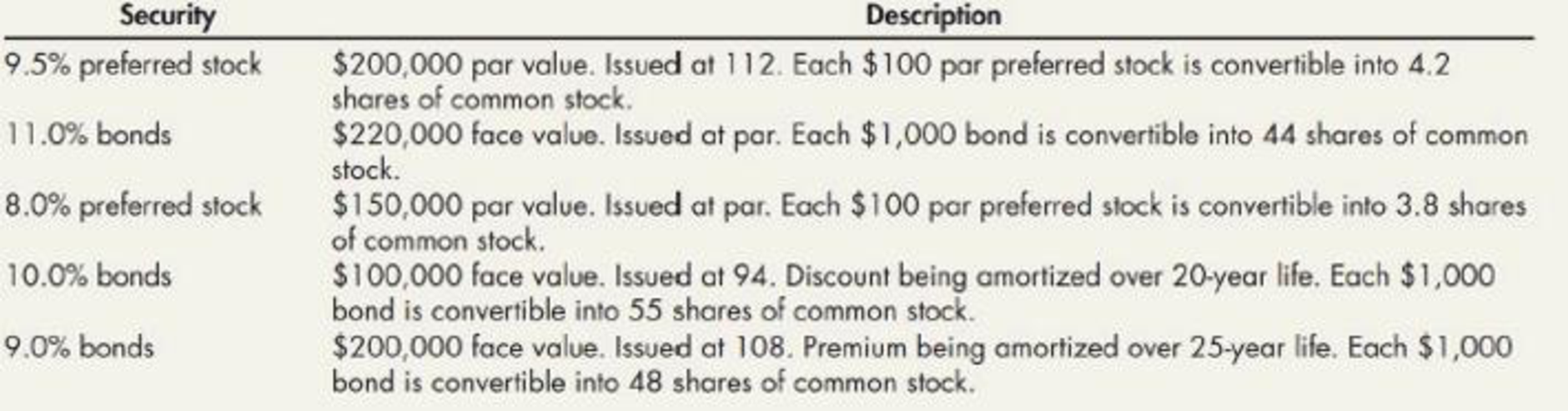Chapter 16, Problem 21E### Intermediate Accounting: Reporting...

3rd Edition
James M. Wahlen + 2 others
ISBN: 9781337788281

#### Solutions

Chapter
Section### Intermediate Accounting: Reporting...

3rd Edition
James M. Wahlen + 2 others
ISBN: 9781337788281
Textbook Problem
1 views

# Mills Company had five convertible securities outstanding during all of 2019. It paid the appropriate interest (and amortized any related premium or discount using the straight-line method) and dividends on each security during 2019. Each convertible security is described in the following table. The corporate income tax rate is 30%.Required: 1. Prepare a schedule that lists the impact of the assumed conversion of each convertible security on diluted earnings per share. 2. Prepare a ranking of the order in which the securities would be included in the diluted earnings per share computations.

1.

To determine

Prepare a schedule to show the impact of the assumed conversion of each convertible security on diluted earnings per share.

Explanation

Earnings per share (EPS):

The amount of net income available to each shareholder per common share outstanding is referred to as earnings per share (EPS).

Prepare a schedule to show the impact of the assumed conversion of each convertible security on diluted earnings per share.

 Convertible security Impact in $9.5% preferred stock (1)$2.26 11.0% bonds (2) $1.76 8.0% preferred stock (3)$2.11 10.0% bonds (6) $1.31 9.0% bonds (7)$1.27

(Table 1)

Working notes:

(1) Calculate the impact of the 9.5% preferred stock on diluted earnings per share.

Diluted earnings per share =Net income  obtained on preferreds stock of  9.5%(Number of shares of preferred stock converted into common stock )=200,000×9.5%2,000×4.2=$19,0008,400=$2.26

(2) Calculate the impact of the 11.0% bonds on diluted earnings per share.

Diluted earnings per share =(Net income obtained on 11.0% bonds×Corporation income tax rate)(Number of shares of bonds convertible into common stock  )=($220,000×11.0%)×(130%)220×4.2=$16,9409,680=$1.75 (3) Calculate the impact of the 8.0% preferred stock on diluted earnings per share. Diluted earnings per share =Net income obtained on preferreds stock of 8.0%(Number of shares of preferred stock converted into common stock )=150,000×8.0%1,500×3.8=$12,0005,700=$2.11 (4) Calculate the discount on amortized bond for 20 year life: Discount on amortized bond for 20 year life} = Carrying value Face value=$100,000×94%$100,000=$6,000

(5) Calculate the impact of the 10

2.

To determine

Show the manner by which the securities that are included in the diluted earnings per share are ranked.

### Still sussing out bartleby?

Check out a sample textbook solution.

See a sample solution

#### The Solution to Your Study Problems

Bartleby provides explanations to thousands of textbook problems written by our experts, many with advanced degrees!

Get Started

#### Provide an example of each.

Foundations of Business (MindTap Course List)

#### TIME FOR A LUMP SUM TO DOUBLE How long will it take 200 to double if it cams the following rates? Compounding o...

Fundamentals of Financial Management, Concise Edition (with Thomson ONE - Business School Edition, 1 term (6 months) Printed Access Card) (MindTap Course List)

#### List and describe six costs of inflation.

Principles of Macroeconomics (MindTap Course List)